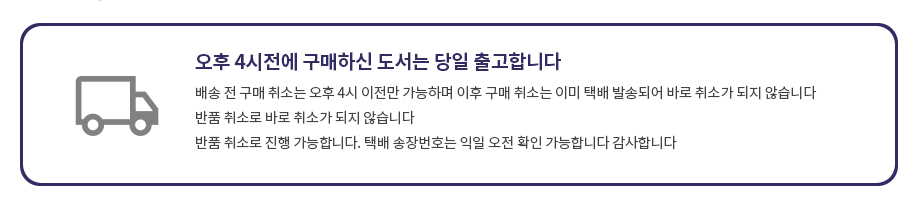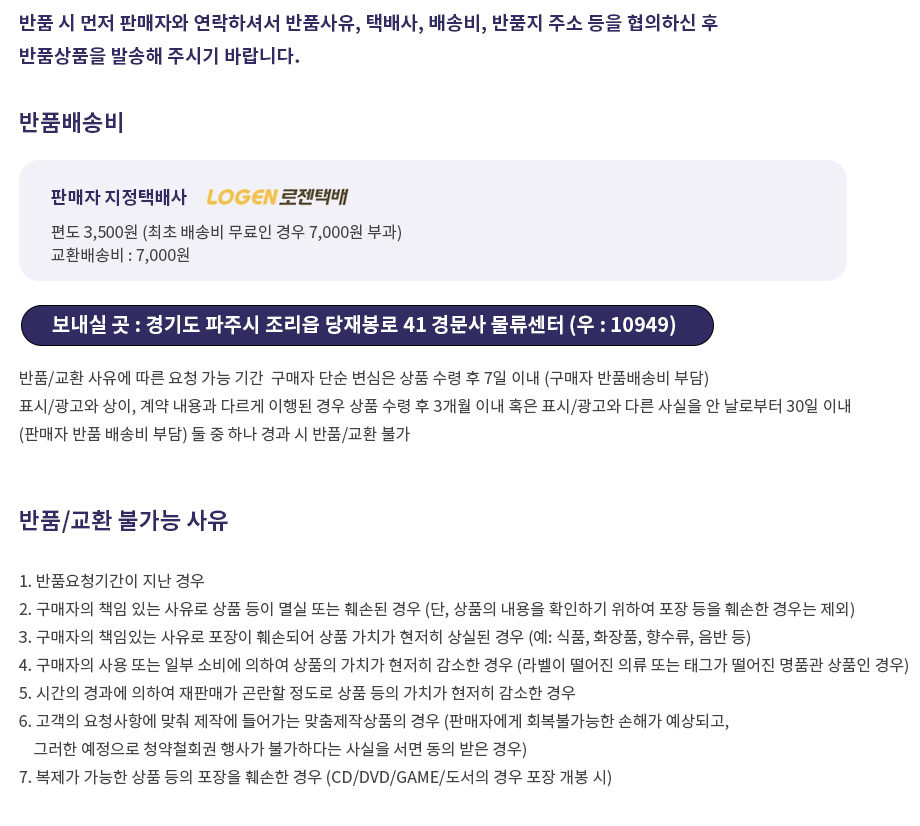## 로그인이필요합니다## 도서를 검색해 주세요.

원하시는 결과가 없으시면 문의 주시거나 다른 검색어를 입력해보세요.

•상품상세

## Basic Engineering Mathematics(2005) 요약정보 및 구매

상품 선택옵션 0 개, 추가옵션 0 개

사용후기 0 개
지은이 Bird 2005-03-05 4판 304 9780750665759 구매가능 37,000원 0점 주문시 결제

### 선택된 옵션

• Basic Engineering Mathematics(2005)
+0원

## 관련상품

• Unlike most engineering maths texts, this book does not assume a firm grasp of GCSE maths, and unlike low-level general maths texts, the content is tailored specifically for the needs of engineers. The result is a unique book written for engineering students, which takes a starting point below GCSE level. Basic Engineering Mathematics is therefore ideal for students of a wide range of abilities, and especially for those who find the theoretical side of mathematics difficult. All students taking vocational engineering courses who require fundamental knowledge of mathematics for engineering and do not have prior knowledge beyond basic school mathematics, will find this book essential reading. The content has been designed primarily to meet the needs of students studying Level 2 courses, including GCSE Engineering and Intermediate GNVQ, and is matched to BTEC First specifications. However Level 3 students will also find this text to be a useful resource for getting to grips with the essential mathematics concepts needed for their study, as the compulsory topics required in BTEC National and AVCE / A Level courses are also addressed. The fourth edition incorporates new material on adding waveforms, graphs with logarithmic scales, and inequalities - key topics needed for GCSE and Level 2 study. John Bird's approach is based on numerous worked examples, supported by 600 worked problems, followed by 1050 further problems within exercises included throughout the text. In addition, 15 Assignments are included at regular intervals. Ideal for use as tests or homework, full solutions to the Assignments are supplied in the accompanying Instructor's Manual, available as a free download for lecturers from http://textbooks.elsevier.com. * Unique in introducing fundamental mathematics from an engineering perspective, with a starting point below GCSE level * Fully matched to BTEC First and BTEC National core unit specifications * Free instructor's manual available to download - contains worked solutions and suggested mark scheme
• 1.Basic aritmetic 2.Fractions,decimals and percentages 3.Indices,standard form and engineering notation 4.Calculations and evaluation of formulae 5.Computer numbering systems 6.Algebra 7.Simple equations 8.Transposition of formulae 9.Simultaneous equations 10.Quadratic equations 11.Inequalities 12.Straight line graphs 13.Graphical solution of equations 14.Logarithms 15.Exponential functions 16.Reduction of non-linear laws to linear-form 17.Graphs with logarithmic scales 18.Geometry and triangles 19.Introduction to trigonometry 20.Trigonometric waveforms 21.Cartesian and polar co-ordinates 22.Areas of plane figures 23.The circle 24.Volumes of common solids 25.Irregular areas and volumes and mean values of waveforms 26.Triangles and some practical applicaions 27.Vectors 28.Adding of waveforms 29.Number sequences 30.Presentation of statistical data 31.Measures of central tendency and dispersion 32.Probability 33.Introduction to differentiation 34.Introduction to integration
• 학습자료

등록된 학습자료가 없습니다.

정오표

등록된 정오표가 없습니다.

사용후기가 없습니다.

상품문의가 없습니다.

• ## 배송/교환정보

### 배송정보### 교환/반품 정보### 선택된 옵션

• Basic Engineering Mathematics(2005)
+0원